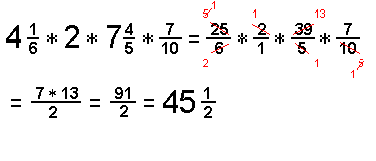# Working with FractionsMultiplying FractionsFollow the following steps to multiply fractions:

1.   Write the numbers horizontally:

Go to the NEXT step...

2.   Are there any Whole or Mixed numbers?

YES:   Continue below...

Change whole or mixed numbers to improper fractions.

• Write a WHOLE NUMBER OVER ONE in the denominator.
(Any number divided by one is still itself.)
• To change a MIXED NUMBER to an improper fraction:
MULTIPLY THE DENOMINATOR
(bottom number = the number of pieces in each whole thing) TIMES THE WHOLE NUMBER;
(top number = the number of extra pieces)
Write this total OVER the original denominator
(the total number of those-sized pieces in all).
• Repeat with the other fraction.

Go to the NEXT step...

3.   Can you Cancel?
YES:   Continue below...

SIMPLIFY: Eliminate common factors.

• Divide ANY single numerator and ANY single denominator by the SAME NUMBER (a common factor);
• Cross out the original and write the new numbers for each (remember to write the amount that's LEFT and not the common factor by which you'd divided).
• Repeat as many times as possible; by sure to use one numerator and one denominator each time.

Go to the NEXT step...

4.   Do the Multiplication:

MULTIPLY ACROSS:

• The PRODUCT of the NUMERATORS is the top number of the answer;
• The PRODUCT of the DENOMINATORS is the bottom number of the answer.

Go to the NEXT step...

5.   Is the Fraction in Lowest Terms?

NO:   Continue below...

Improper fraction:   (Top number is greater than the bottom number.)
• Divide the top BY the BOTTOM;
• Write any remainder as the numerator and use the same denominator.

Simplify the fraction:
• Divide both the numerator and the denominator by the same number.
• Repeat until the only common factor is one.

Go to the NEXT step...

SAMPLE PROBLEM #1:

2/7 * 4/5 =

Solution: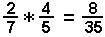SAMPLE PROBLEM #2:

5/12 * 9/10 =

Step 1: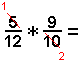Step 2: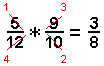SAMPLE PROBLEM #3:

25/8 * 6 =

Initial Setup: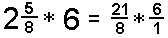Step 1: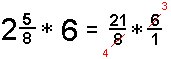Step 2: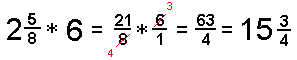SAMPLE PROBLEM #4:

4 1/6 * 2 * 7 4/5 * 7/10 =

Initial Setup:Step 1: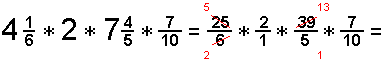Step 2: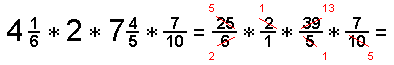Step 3: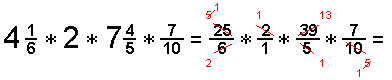Solution - Part 1:Solution - Part 2: